##1. A property of trapezia

Consider a trapezium ABCD with diagonals {AC, BD}. From the end-points of the first diagonal draw two parallels {e,e'} and from the end-points of the other diagonal draw two other parallels {f,f'}. Let E  be the intersection of lines {e',f} and F the intersection of lines {e,f'}. Then triangles EAD and FBC on the non-parallel sides of the trapezium have equal areas.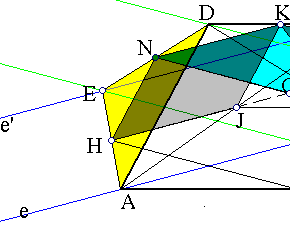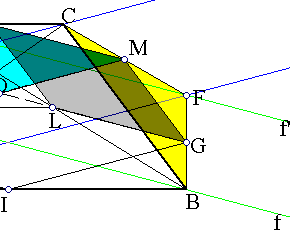To prove it take everywhere the middles : {H, N, K, M, G, L, J} respectively of {EA, ED, DC,
CF, FB, BD, AC}. The quadrilaterals q1=ACDE and q2=BDCF have their parallelograms of the middles of sides {HJKN, LGMK} respectively of equal areas. In fact, both are equal in area to the parallelogram OMKN, where O is the intersection of lines {GN, HM}. Consequently {q1, q2} have the same area. But their area is ADE+ACD = BFC+BCD and ACD=BCD, hence ADE=BFC (equality here meaning area-equality).
Remark Notice that this simple property gives a geometric proof of the fact that every non-involutive affinity preserving a central conic is an equi-affinity i.e. it is a map preserving areas [PamfilosBitangent, p. 256 ]).

##2. Some consequences

With the settings of the previous paragraph:
 Lines {EM, FM} which are parallel to {AD, BC} from {E,F} respectively, intersect at a point M on line KI joining the middles of the parallel sides of the trapezium.
 Let {E',F'} be the projections of {E, F} on sides {AD, BC} respectively. The ratio EE'/FF' = BC/AD, thus it is constant and independent of the particular direction of the parallels {e,e',f,f'}.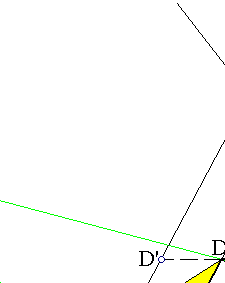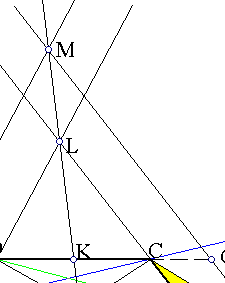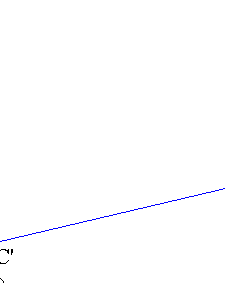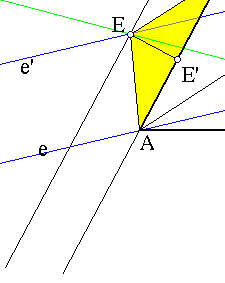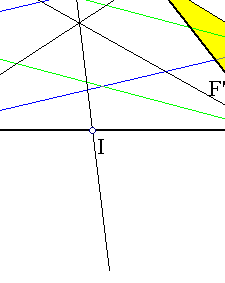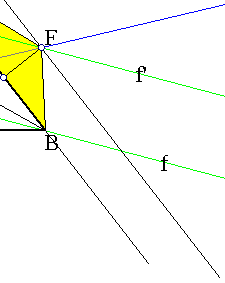Follows immediately from the preceding paragraph.  follows from . In fact, extend DC until to intersect the parallels at {D',C'}. Then DD'=EE'/sin(D) and CC'=FF'/sin(C). Dividing the terms and using the parallelity from which sin(C)/sin(D)=sin(B)/sin(A)=AD/BC, follows that DD'=CC'. Thus {D',C'} are symmetric with respect to K and  consequently M is on LK.